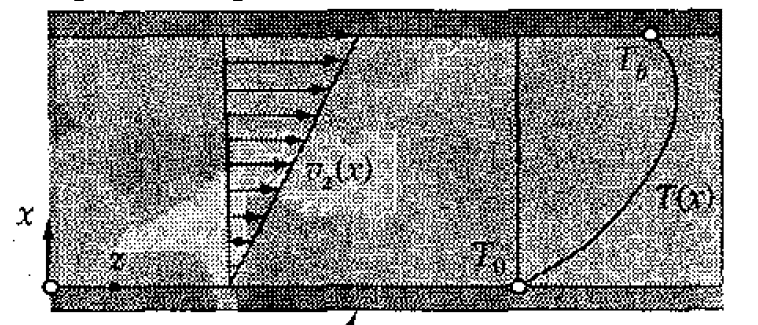# Viscous work and dissipation of energy

• Est120
In summary, the conversation discusses the concept of work being done on a fluid system by an external source, such as an upper plate moving with speed v in the +z direction. It is determined that in this scenario, both the fluid and surroundings are doing work on each other. The signs convention is taken into account to show that the internal energy of the system increases when work is done on it by the surroundings, and decreases when the system does work on the surroundings. It is also noted that when both the system and surroundings are doing work on each other, there is no net work inflow into the system and the internal energy remains constant.f

#### Est120

TL;DR Summary
viscous dissipation of mechanical energy into internal energy
imagine two flat plates, the upper one moving with speed v in the +z direction, the lower one is stationary
is the upper plate inserting work (per unit time) on the fluid system or the system exerting work on the plate?
or both? why they don't cancel each other e.g if the fluid is receiving work from the upper plate then its internal energy is increasing, but if the fluid exerts work too then the works cancel (?)then the works cancel
In what sense ? Do you have the same problem with a block that is dragged over a surface ?

•russ_watters
Would the molecules of the viscous fluid move without the movement of the upper plate?
If yes, the fluid would be receiving energy from an external source (pump or gravity), which would be transferred onto the upper plate.
If not, the upper plate would be receiving energy from an external source (force along z-axis) which would be transferred into the fluid.

the fluid is moving because of not split condition with the upper plate so its the second case

•Lnewqban
in this case, the surroundings are doing work on the fluid. The force of the surrounding and its displacement are both positive. The fluid is doing negative work on the surroundings (the force of the fluid and its displacement are in opposite directions). So, if you write ##\Delta U=Q-W##, where W is the work the fluid does on the surroundings, or ##\Delta U=Q+W##, where W is the work done by the surroundings of the fluid, the result is exactly the same; the internal energy increases.

in this case, the surroundings are doing work on the fluid. The force of the surrounding and its displacement are both positive. The fluid is doing negative work on the surroundings (the force of the fluid and its displacement are in opposite directions). So, if you write ##\Delta U=Q-W##, where W is the work the fluid does on the surroundings, or ##\Delta U=Q+W##, where W is the work done by the surroundings of the fluid, the result is exactly the same; the internal energy increases.
so that's why the work doesn't cancel? the signs convention already takes into account the dual work issue (work enviroment→ system and work system→enviroment)

so that's why the work doesn't cancel? the signs convention already takes into account the dual work issue (work enviroment→ system and work system→enviroment)
It doesn't cancel for the same reason that, if A is exerting a force on B, and B is exerting an equal and opposite reaction force on A, if you do a force balance on A, you only include the force that B exerts on A, not the force that A exerts on B. Didn't they teach you this in freshman Physics?

i know that... but it seems no body understands my doubt
if the environment is exerting work on the system, then the system internal energy ↑ increases
but if the system is exerting work on the environment then, energy must come out of the system (in order to deliever work) and that energy decreases internal energy ↓ so internal energy decreases
so if the system is receiving work and also delivering work no net work inflow comes into the system so internal energy stays constant

i know that internal energy must increase but i don't know what i am thinking wrong about work

•weirdoguy
i know that... but it seems no body understands my doubt
if the environment is exerting work on the system, then the system internal energy ↑ increases
but if the system is exerting work on the environment then, energy must come out of the system (in order to deliever work) and that energy decreases internal energy ↓ so internal energy decreases
so if the system is receiving work and also delivering work no net work inflow comes into the system so internal energy stays constant

i know that internal energy must increase but i don't know what i am thinking wrong about work
Apparently, you don't understand what I said. If the system does positive work on the surroundings, then the surroundings do a negative amount of work on the system, so the two are equivalent. But, if you are doing an energy balance on the system, then you only include one of these, just as, when you do a force balance, you only include one force of the reaction pair.

i got it now, thank's for all the advice

•Lnewqban